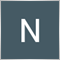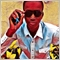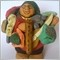Error lots constant cannot be modified.... I need help with this code14

//+------------------------------------------------------------------+
//|                                                                 . |
//|                                                                   |
//+------------------------------------------------------------------+
#property version   "1.00"
#property strict

input double lots =0.1;
input int stoploss= 30;
input int takeprofit= 40;
input int magic1=302;
input int slippage= 3;
input double lotfactor= 0.50;
input int totalorders=8;
input int EnterHour =4;

//+------------------------------------------------------------------+
{
lots+=lotfactor;
}
{
int ticket, expiration;
double point;
//----
point=MarketInfo(Symbol(),MODE_POINT);
expiration=NULL;
//----
while(true)
{
if(ticket<=0)Print("Error = ", GetLastError());
else{Print("ticket = ", ticket); break; }
//---- 10 seconds wait
Sleep(10000);
}
lots+=lotfactor;
}

void opensell()
{
lots+=lotfactor;
}
void opensellstop()
{
int ticket, expiration;
double point;
//----
point=MarketInfo(Symbol(),MODE_POINT);
expiration=NULL;
double price = Bid-50*Point;
//----
while(true)
{
if(ticket<=0)Print("Error = ", GetLastError());
else{Print("ticket = ", ticket); break; }
//---- 10 seconds wait
Sleep(10000);
}
lots+=lotfactor;
}

int countpending()
{
int s=0;
for(int i=0;i<OrdersTotal();i++)
{
if(OrderType() > OP_SELL)
{
s++;
}

}
return(s);
}
void closeallorders()
{
for(int i=0;i<OrdersTotal();i++)
{
{
int j=0;
while(j!=5)//try 5 times to ensure execution
{
OrderClose(OrderTicket(),OrderLots(),Bid,slippage,Pink);
j++;
}
}
}
}
Files:
complain1.txt  3 kb12119

extern double lots =0.1;4961

Nafisat Lateef:

Is not a good practise changing the input parameters because once changed you cannot reset the value until the EA is restarted.

input double inplots =0.1;
input int stoploss= 30;
input int takeprofit= 40;
input int magic1=302;
input int slippage= 3;
input double lotfactor= 0.50;
input int totalorders=8;
input int EnterHour =4;

double lots = inplots;

//+------------------------------------------------------------------+
{
lots+=lotfactor;
}
{
int ticket, expiration;
double point;
//----
point=MarketInfo(Symbol(),MODE_POINT);
expiration=NULL;
//----
while(true)
{
if(ticket<=0)Print("Error = ", GetLastError());
else{Print("ticket = ", ticket); break; }
//---- 10 seconds wait
Sleep(10000);
}
lots+=lotfactor;
}

void opensell()
{
lots+=lotfactor;
}
void opensellstop()
{
int ticket, expiration;
double point;
//----
point=MarketInfo(Symbol(),MODE_POINT);
expiration=NULL;
double price = Bid-50*Point;
//----
while(true)
{
if(ticket<=0)Print("Error = ", GetLastError());
else{Print("ticket = ", ticket); break; }
//---- 10 seconds wait
Sleep(10000);
}
lots+=lotfactor;
}9

Fernando Morales #:

Is not a good practise changing the input parameters because once changed you cannot reset the value until the EA is restarted.

So easy!Thank you so much, amigo!)26723